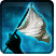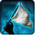# [report_difficulty]Conditions

## Related effects, buffs and debuffs

• [0s] [not displayed]Effect #1

 Slot: Buff Duration: 0 Sek. Tick rate: does not tick # occurrences: 0
• On Apply

Only when the following conditions are met:

• If difficulty is set to 8-man SM

Perform the following actions:

• Custom
- Param 2 = (string) DIFFICULTY 1
- Param 3 = (string)
- Param 4 = (string)
- Param 5 = (string)
- Param 6 = (string)
- Param 7 = (string)
- Param 8 = (string)
- Param 9 = (string)
- Param 1 = (int) 9499481598613081908
• On Apply

Only when the following conditions are met:

• If difficulty is set to 8-man HM

Perform the following actions:

• Custom
- Param 2 = (string) DIFFICULTY 2
- Param 3 = (string)
- Param 4 = (string)
- Param 5 = (string)
- Param 6 = (string)
- Param 7 = (string)
- Param 8 = (string)
- Param 9 = (string)
- Param 1 = (int) 9499481598613081908
• On Apply

Only when the following conditions are met:

• If difficulty is set to 16-man SM

Perform the following actions:

• Custom
- Param 2 = (string) DIFFICULTY 3
- Param 3 = (string)
- Param 4 = (string)
- Param 5 = (string)
- Param 6 = (string)
- Param 7 = (string)
- Param 8 = (string)
- Param 9 = (string)
- Param 1 = (int) 9499481598613081908
• On Apply

Only when the following conditions are met:

• If difficulty is set to 16-man HM

Perform the following actions:

• Custom
- Param 2 = (string) DIFFICULTY 4
- Param 3 = (string)
- Param 4 = (string)
- Param 5 = (string)
- Param 6 = (string)
- Param 7 = (string)
- Param 8 = (string)
- Param 9 = (string)
- Param 1 = (int) 9499481598613081908
• On Apply

Only when the following conditions are met:

• If difficulty is any of

Perform the following actions:

• Custom
- Param 2 = (string) DIFFICULTY 5???
- Param 3 = (string)
- Param 4 = (string)
- Param 5 = (string)
- Param 6 = (string)
- Param 7 = (string)
- Param 8 = (string)
- Param 9 = (string)
- Param 1 = (int) 9499481598613081908
• On Apply

Only when the following conditions are met:

• If difficulty is any of 8-man SM, 8-man HM, 16-man SM, 16-man HM

Perform the following actions:

• Custom